Warning: count(): Parameter must be an array or an object that implements Countable in /home/customer/www/thegatebook.in/public_html/qa/qa-include/qa-theme-base.php on line 177

# DM - Grand Test -Q6

Warning: count(): Parameter must be an array or an object that implements Countable in /home/customer/www/thegatebook.in/public_html/qa/qa-include/qa-theme-base.php on line 177
+1 vote

A latticeis called a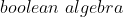if it is both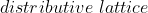and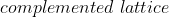. Which of the following statement is false in case of a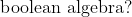(A) In aevery element will have a unique complement.

(B) The poset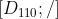is a boolean algebra , where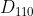is the set of all divisors of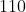andrepresents the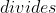relation.

(C) Let A=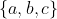and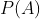represents the power set of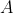, then the poset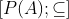where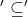represents the subset relation is a boolean algebra.

(D) Every total ordered relation is a boolean algebra.asked Aug 30, 2020
reshown Aug 31, 2020

A TOS on n elements is BA iff n=2. So, Option D is false.

In a distributive lattice each element has atmost one complement (that is either no complements or 1 complement.

In a lattice if upper bound and lower bound exists then it is called a bounded lattice. Let L be a bounded lattice, if each element of L has complement in L , then L is called a complemented lattice. In a complemented lattice each element has atleast one complement. A lattice is boolean algebra if it is both distributive and complemented. So in a boolean algebra each element will have exactly one complement.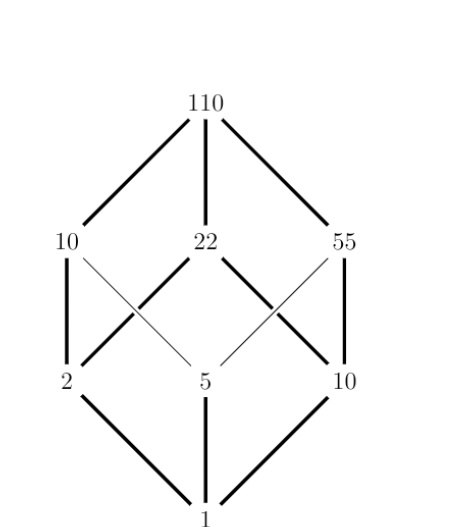The hasse diagram is identical to hasse diagram for the poset So its a boolean algebra. Complement of 10 is 11, Complement of 110 is 1, Complement of 22 is 5, Complement of 55 is 2, And vice - versa.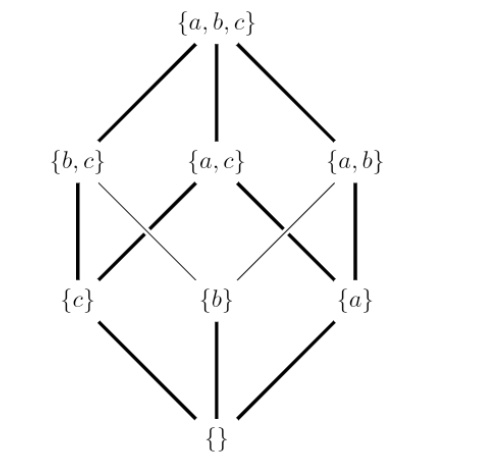Every total ordered relation is distributive but a total order relation which contain more than 2 elements cant be complemented since in a total ordered chain elements are directly related , so we wont get complements for elements other than Upper bound and lower bound.

Option D is the correct answeranswered Sep 9, 2020 by (226,240 points)
amazing explanation, with crystal clear concepts.